# Data Jobs: What does a Data Analyst Do?

Data Analysts get a bad wrap. With the advent of the Data Scientist, Data Analysts are often viewed as Data Scientists lite, however I feel that is not the honest case. Truth is, there is a lot of overlap between the two fields. I will dive deeper into what a Data Scientist is in a future article, but just know my opinion is the definition of Data Scientist as a job is still a bit fuzzy and I think the job title may eventually be broken into a few different titles to better define the differences.

## Data Analyst

So what does a Data Analyst do?

A lot actually. You could put 10 data analysts into a room and you would get ten different answers to this question. So the best I can do here is make sweeping generalities. As the old saying goes “Your results may vary”

In general, data analysts perform statistical analysis, create reporting, run ad-hoc queries from data warehouses, create data visualizations, create and maintain dashboards, perform data mining, and create machine learning models (yes, ML is not only for data scientists). Their assignments are business driven. A data analysts is either embedded with a business unit (financial planning, fraud, risk management, cyber security, etc.) or working in a centralized reporting/analytics team. They use their skills to provide reporting and analytics for the business.

## Tools used by Data Analysts

• SQL – MySql, SQL Server, Oracle, Teradata, Postgres – whether simply querying a data warehouse or creating and managing a local data mart, data analysts need to be advanced SQL programmers
• Visualization tools – Tableau, Qlik, Power BI, Excel, analysts use these tools to create visualizations and dashboards
• Python/R – Data analysts should be familiar with languages like Python or R to help manage data and perform statistical analysis or build machine learning models
• Spreadsheets – Excel, Google Sheets, Smart Sheets are used to create reports, and pivot tables used to analyze the data
• ETL tools – SSIS, Alteryx, Talend, Knime, these tools are design to move data to and from databases, CSV files, and spreadsheets. Until the data is in a usable format, analysis cannot be performed.

## Educational Requirements

Typically a data analyst position will ask for a bachelors degrees, preferably in computer science, statistics, database management or even business. While the barrier to entry for a data analyst job is generally not as high as a data scientist, that does not mean you cannot make a meaningful and well paid career as a data analyst. Also, the demand for data professionals seems to keep going up and up and it most likely will for the foreseeable future.

# How do I get a job in Data Science?

This has to be the most common question on data science I am asked, and honestly it is a hard one to answer. For everyone out there trying to get your foot in the door on your first data job, believe me, I feel for you. Multiple interviews without any offers, or even not getting any interviews at all can be beyond frustrating. Now unfortunately, I do not have any magic trick to get your into the data field, but I can share how I did it.

So, how did I get into the data science field…

Honestly, I “Made” my first job. My first career out of the Army was as a biomedical equipment technician. I fixed medical equipment like patient monitors, ultrasounds, and x-ray machines.

We had a ticketing system called MediMizer where all the repairs and routine maintenance jobs were recorded. My bosses would run monthly reports out the system. I read some of the reports and just felt like we could do better.

I started with just Excel. I downloaded some data, created some pivot charts and made some basic visualizations. I asked new questions from the data. I looked at angles that weren’t covered in the existing reporting.

I showed these to my bosses, my co-workers, other department managers, basically anyone who would listen to me. Then I learned about Tableau, and using its free version I was able to create some more professional looking visualizations.

I learned how to make a dashboard, I started analyzing data sets from other departments, and I began feeding them my reports. I went back to school to get a degree and used what I was learning in school to improve my reporting skills.

While my job title didn’t change, I was now able to put data analysis skills on my resume. I was lucky enough to have very supportive management who saw the value in what I was doing, and allowed me to dedicate some of my time to it.

But most importantly, I was now a data professional (even if not in title). I was using data to solve real world problems. I put together a portfolio of some of the reporting I was doing. This allowed me to show my future employer that not only was I able to create reporting, but more importantly I was able to identify real world business problems and use data to help solve them.

The take away is don’t let your job title hold you back. Look around, what kind of problems do you see? Can you find a data-driven solution to help fix the problem? If you do this, you are a now a data professional (even if not in title). A portfolio made from real world examples can be more impressive than generic tutorial or Kaggle projects.

Remember, when trying to break into a new field, sometimes you need to make your own luck.

# R Shiny: Introduction

Shiny is an R Studio application that allows you to how host your R code on a website. This is a great method for creating a dashboard or deploying a predictive model. The best part is, it is free. There are, however, paid services associated with R Studio and Shiny if you want to get professional support and such.

In this lesson, we are going to learn to build a Shiny server application step by step.

Let’s start at the beginning. First off, to use Shiny, you need to have R Studio installed on your computer. Once you have that, you will need to install the Shiny package

install.packages(‘shiny’)

Next, we are going to learn about the three parts of a Shiny server application.

Below is the basic framework of a Shiny app:

```library(shiny)

ui <- fluidPage("Test")
server <- function(input, output) {}
shinyApp(ui = ui, server = server)```

Let’s break this down:

ui: ui is the user interface. This is the code that makes up the web page the user will launch and interact with. Anyone familiar with HTML, this is the basic markup. This is where you set the wording, font, colors, what kind of object will be on the page, etc

server: server is the brains of the operation. This is where you load and manipulate any data being used in your page. Again, if you are a web developer, think Java Script here.

shinyApp: this is the code that launches your App.

If you run the code above, you will see something like this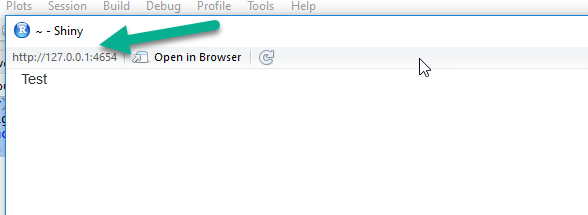Note the IP address in the upper left. If you copy this into a browser of your choice, it will run there too.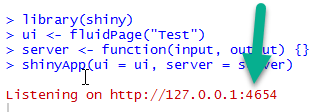You will see the same address in the R Console. As long as the R Shiny server is running, you will see this Listening command. For those not familiar, the address 127.0.0.1 is called a pingback address. This is the internal address of the NIC card on your machine. This means the server is running on the machine you are on. The :4654 is the port my computer grabbed. This can be different for you. The easiest way for me to explain the port without going too deep into network theory is: think of it like a TV channel. From your computer, you could technically be running hundreds of these R Shiny servers. The port (TV channel) is what tells the computer which one of these web pages you want to visit.

If you happen to be on a network, you can go to another computer and type in your host computers network IP address (you can get it by going to a cmd console and typing ipconfig). It will be the address I have the arrows pointing to in my exampleThen, using my example, type http://10.171.46.91:4654 into the second computer’s browser and you should see your Shiny website.

### Step 2

Let’s see if we can make our website more interesting, let’s start easy, we will format the text.

First close out your shiny window to end the session.

Change your ui line to match the one below.

ui <- fluidPage(h1(“Test”))

library(shiny)
ui <- fluidPage(h1(“Test”))
server <- function(input, output) {}
shinyApp(ui = ui, server = server)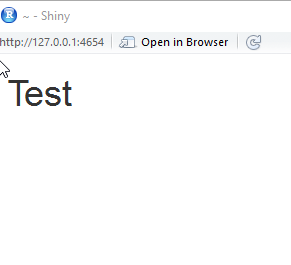### Step 3

Okay, let’s make it a little more interesting by adding some detail to our page. We will do so by using panel methods in our fluidPage()

```library(shiny)

ui <- fluidPage(
titlePanel("Title Panel"),
sidebarPanel("Sidebar Panel"),
mainPanel("Main Panel")
)
server <- function(input, output) {}
shinyApp(ui = ui, server = server)```

Notice your page is now segmented into a title bar, a sidebar and a main panelOkay, now let’s actually add some R data magic to this.

```library(shiny)
library(datasets)
mpgData <- mtcars

ui <- fluidPage(
titlePanel("Mpg vs Cylinder Plot"),
sidebarPanel("Sidebar Panel"),
mainPanel(  plotOutput("mpgPlot"))
)
server <- function(input, output) {
output\$mpgPlot <- renderPlot({
boxplot(mpg~cyl, data= mpgData)})
}
shinyApp(ui = ui, server = server)```

Let’s break down the new code. First thing first, we are loading the built in datasets library and grabbing the mtcars data set from it.

library(datasets)

mpgData <- mtcars

Next we are going to skip down to the server section next as this is where we work with our data:

First thing you will notice is function(input, output) – this is a standard call that allows you to pass information back and forth through the server code. In our example, we are only using the output right now.

We create a variable called output\$mpgPlot to which we pass the shiny method renderPlot.  Inside that method, we are simply creating a simple boxplot comparing mpg to cyl using the mtcars data set that we labeled mpgData

```server <- function(input, output) {
output\$mpgPlot <- renderPlot({
boxplot(mpg~cyl, data= mpgData)})
}```

The boxplot we rendered as output\$mpgPlot is now passed to our ui

Next, in the ui I renamed the title panel and in the mainPanel, I added the command plotOutput(“mpgData”). That will allow me to post the boxplot I created in the server to the ui web page.

```ui <- fluidPage(
titlePanel("Mpg vs Cylinder Plot"),
sidebarPanel("Sidebar Panel"),
mainPanel(  plotOutput("mpgPlot"))
)```

If you run the code, it should look like this: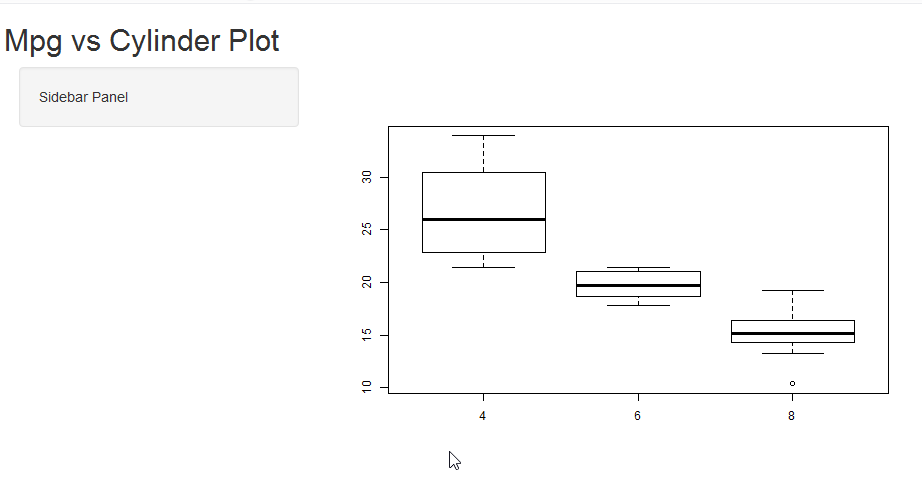### Step 4

Now let’s add a little interactivity to our web page. We are going to give the user the option to view the boxplots with or without the outliers taken into effect.

```library(shiny)
library(datasets)
mpgData <- mtcars

ui <- fluidPage(
titlePanel("Mpg vs Cylinder Plot"),
sidebarPanel(checkboxInput("outliers", "Show outliers", FALSE)),
mainPanel(
plotOutput("mpgPlot"))
)
server <- function(input, output) {
output\$mpgPlot <- renderPlot({
boxplot(mpg~cyl, data= mpgData,
outline = input\$outliers)})
}
shinyApp(ui = ui, server = server)```

This involves only two changes to our code

First we will add a checkbox to our sidebar, in the ui we will alter the sidebarPanel line to look like this

sidebarPanel(checkboxInput(“outliers”, “Show outliers”, FALSE)),

The breakdown of the syntax here is

checkboxinput(variable name, display text, default )– our default is set to FALSE so our box will be unchecked by default

Next, we will alter our boxplot to take into account the checkbox input

boxplot(mpg~cyl, data= mpgData,
outline = input\$outliers)

— notice the variable name is input\$outliers  It is a composite of the variable from our checkboxinput method and “input” from out function(input, output) function that opens up our server.

UncheckedChecked# Python: An Interesting Problem with Pandas

I was writing a little tongue and cheek article for LinkedIn on fraud detection using frequency distributions (you can read the article here: LinkedIn). While this was a non-technical article, I wanted to use some histograms from a real data set, so I uploaded a spread sheet into Python and went to work.

While working with the data I ran into an interesting problem that had me chasing my tail for about 10 minutes before I figured it out. It is a fun little problem involving Series and Dataframes.

As always, you can upload the data set here: FraudCheck1

```import pandas as pd
("C:\\Users\\Benjamin\\OneDrive\\Documents\\article\\python\\FraudCheck1.xlsx")The data is pretty simple here. We are concerned with our answer column and the CreatedBy (which is the employee ID).  What I am trying to do is see if the “answer”  (a reading from an electric meter) are really random or if they have been contrived by someone trying to fake the data.

First, I want to get the readings for all the employees, so I used pop() to place the answer column into a separate list.

```df1 = df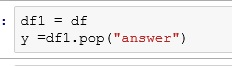Then, to make my histogram more pleasant looking, I decided to only use the last digit before the decimal. That way I will have 10 bars (0-9). (Remember, this is solely for making charts for an article. So I was not concerned with any more stringent methods of normalization)

What I am doing below is int(199.7%10). Remember % is the modulus – leaves you with the remainder and int converts your float to an integers. So 199.7 is cut to 199. The 199/10 remainder = 9.

```a= []
i = 0
while i < len(y):
a.append(int(y[i]%10))
i += 1
a[1:10]```Then I created my histogram.

```%matplotlib inline
from matplotlib import pyplot as plt
plt.hist(a)```## Now my problem

Now I want graph only the answers from employee 619, so first I filter out all rows but the ones for employee 619.

```df2 = df.query('CreatedBy == 619')

Then I ran my loop to turn my answers into a single digit.

And I get an error.  Why?Well the answer lies in the datatypes we are working with. Pandas read_excel function creates a Dataframe.

When you pop a column from a dataframe, you end up with a Series. And remember a series is an indexed listing of values.

Let’s look at our Series. Check out the point my line is pointing to below. Notice how my index jumps from 31 to 62. My while loop counts by 1, so after 31, I went looking for y1 and it doesn’t exist.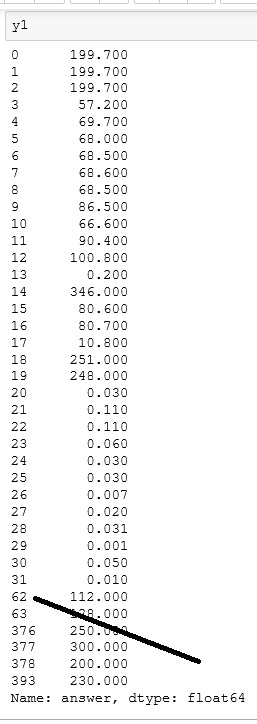Using .tolist() converts our Series to a list and now our while loop works.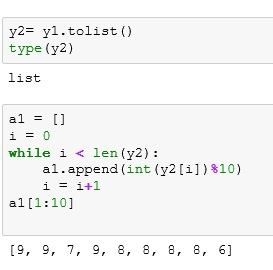And now we can build another histogram.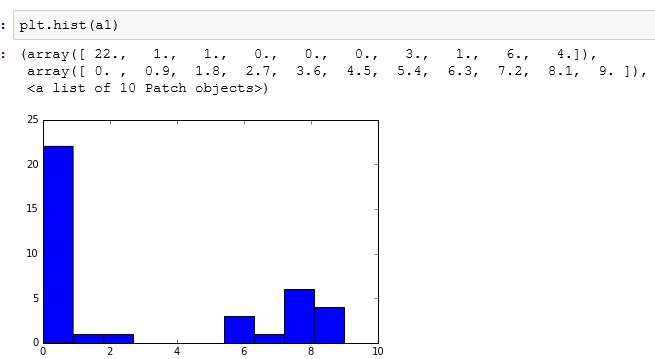## The Code

```import pandas as pd
("C:\\Users\\Benjamin\\OneDrive\\Documents\\article\\python\\FraudCheck1.xlsx")

df1 = df

a= []
i = 0
while i < len(y):
a.append(int(y[i]%10))
i += 1
a[1:10]

%matplotlib inline
from matplotlib import pyplot as plta1 = []
i = 0
while i < len(y2):
a1.append(int(y2[i])%10)
i = i+1
a1[1:10]

plt.hist(a)

df2 = df.query('CreatedBy == 619')

y2= y1.tolist()
type(y2)

a1 = []
i = 0
while i < len(y2):
a1.append(int(y2[i])%10)
i = i+1
a1[1:10]

plt.hist(a1)

```

# Probability vs Odds

Probability and odds are constantly being misused. Even in respected publications you will see sentences such as: “The odds of a Red Sox win tonight is 60%.” or “His probability is 2 to 1.” While in the lexicon of American English these words seem to have taken on interchanging meaning, when working with statisticians or data scientists, you are going to want to get your vocabulary straight.

## Probability:

Probability is a number between 0 and 1, often represented as a fraction or percentage. The probability is determined by dividing the number of positive outcomes by the total number of possible outcomes. So if you have 4 doors and 1 has a prize behind it, your probability of picking the right door is 1/4 or 0.25 or 25%.

Note, do not let the term positive outcome confuse you. It is not a qualifier of good vs bad or happy vs sad. It simply means the result is what you are looking for based on the framing of your question. If I were to state that out of every 6 patients who opt for this new surgery 2 die – 2 would be the “positive outcome” in my equation (2/6 or approx 33%) even though dying is far from a “positive outcome”.

## Odds:

Odds, on the other hand, are a ratio. The odds of rolling a 4 on a six sided die is 1:5 (read 1 in 5). The odds ratio works like this: positive outcomes : negative outcomes. So the odds of rolling an even number on a six sided die is 3:3 (or simplified to 1:1).

Now the probability of rolling an even number on a six sided die is 3/6 or 1/2. So keep that in mind, odds of 1:2 is actually a probability of 1/3 not 1/2.

### Deck of Cards:

Working with a standard deck of playing cards (52 cards).

Pulling a Red card from the deck

• probability: 26/52 = 1/2
• odds: 26:26 = 1:1

Pulling an Ace from the deck

• probability: 4/52 = 1/13
• odds: 4:48 = 1:12

Pulling a Diamond from the deck

• probability: 13/52 = 1/4
• odds: 13:39 = 1:3

# R: Intro to qplot()

qplot() stands for Quick Plot. It is an easy to use plotting tool for R. In order to use qplot(), you need to have ggplot2 installed. To see if you have it, just type the following:

`library(ggplot2)`

If you get an error, you will need to install the package. To install package:

```install.packages("ggplot2")

library(ggplot2)```

Okay, now let’s get some data. R comes will a great collection of data sets you can work with. You can search Google for R data sets. A great source is also the following webpage: https://stat.ethz.ch/R-manual/R-devel/library/datasets/html/00Index.html

I have decided for this exercise to use the ChickWeight data set listed below.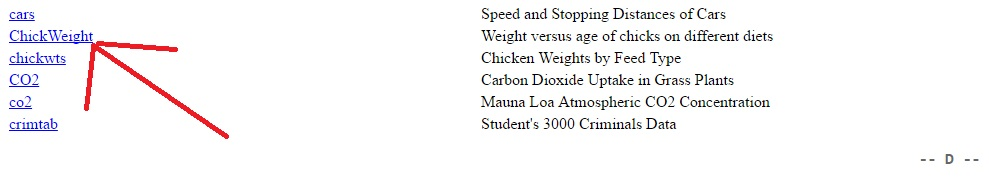To access the dataset in R, simply use the name.

As you can see, the data set contains 4 columns, weight, Time, Chick, Diet

`head(ChickWeight)`Using qplot(), we can get right down to plotting. The basic syntax is: qplot(data, x value)

`qplot(data=ChickWeight, x = weight)`

Notice qplot() automatically labeled our axis. It even created automatic bins for us.`qplot(data=ChickWeight, x = weight, y = Time)`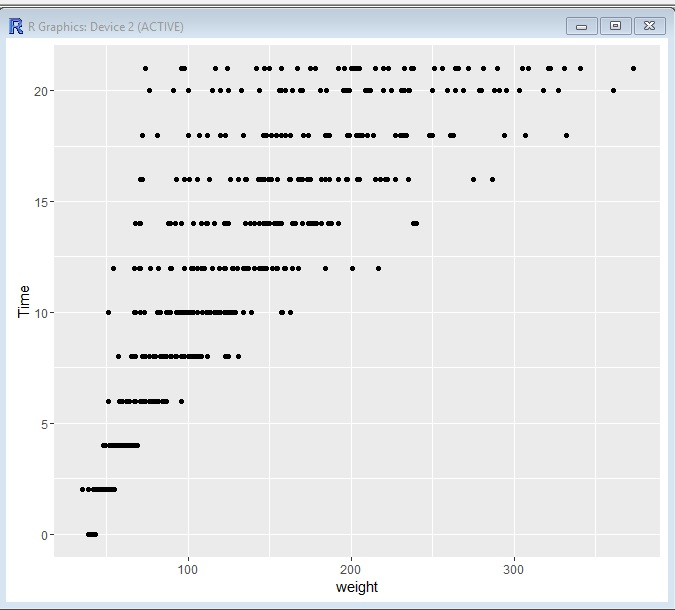Let’s add Diets to the mix now.

`qplot(data=ChickWeight, x= weight, y = Time, color =Diet)`

What jumps out at me right away is the fact the Diet 1 always seems to trail in weight, while Diet 4 seems to lead in weight gain in the earlier weeks, but it is overtaken by 2 and 3 starting around week 12.Now let us add a size element to our graph

`qplot(data=ChickWeight, x= Time, y = Chick,size=weight, color =Diet)`

Now we have Chick’s on the y axis, Time on the X, the size of the dot correlates to weight and the color is diet.

The first thing I notice now is that our data is skewed. We have a lot more chicks on diet 1 than on the other 3 diets. That could effect how we read this data.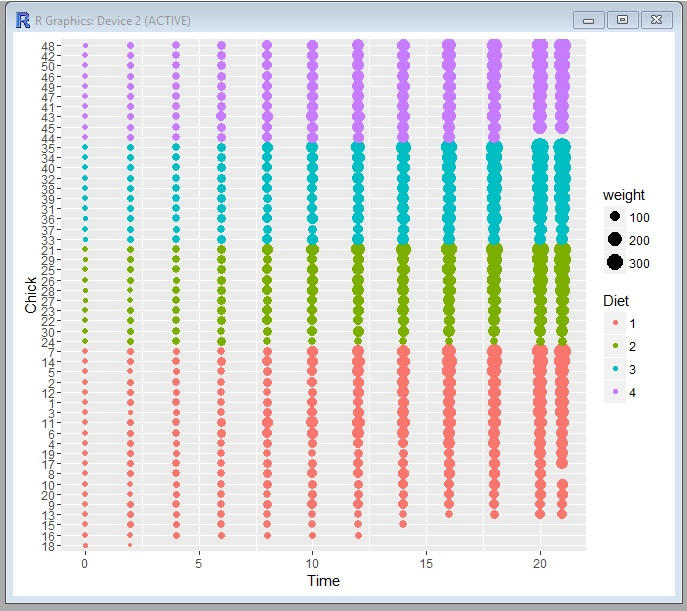And finally, one more just for fun.

`qplot(data=ChickWeight, x= weight, y = Chick, color =Diet)`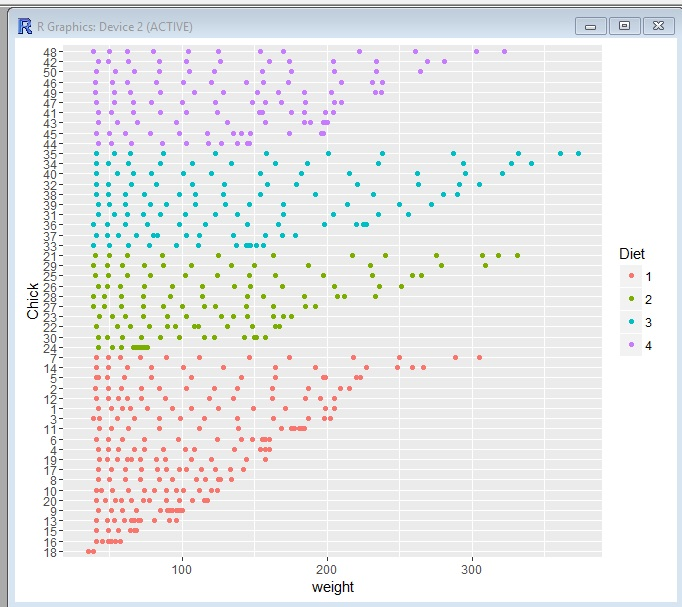# Python: Logistic Regression

This lesson will focus more on performing a Logistic Regression in Python. If you are unfamiliar with Logistic Regression, check out my earlier lesson: Logistic Regression with Gretl

## Import the Data

You should be good at this by now, use Pandas .read_excel().

df.head() gives us a the first 5 rows.

What we have here is a list of students applying to a school. They have a Score that runs from 0 -1600,  ExtraCir (extracurricular activity) 0 = no 1 = yes, and finally Accepted 0 = no 1 = yes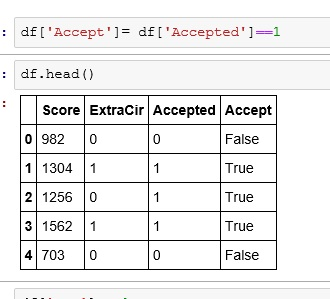## Create Boolean Result

We are going to create a True/False column for our dataframe.

What I did was:

• df[‘Accept’]   — create a new column named Accept
• df[‘Accepted’]==1  — if my Accepted column is 1 then True, else False## What are we modeling?

The goal of our model is going to be to predict and output – whether or not someone gets Accepted based on some input – Score, ExtraCir.

So we feed our model 2 input (independent)  variables and 1 result (dependent) variable. The model then gives us coefficients. We place these coefficients(c,c1,c2) in the following formula.

y = c + c1*Score + c2*ExtraCir

Note the first c in our equation is by itself. If you think back to the basic linear equation (y= mx +b), the first c is b or the y intercept. The Python package we are going to be using to find our coefficients requires us to have a place holder for our y intercept. So, let’s do that real quick.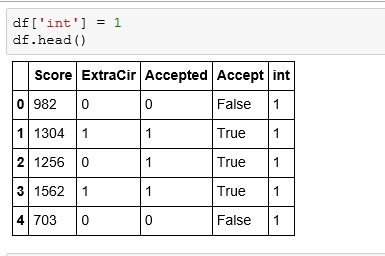## Let’s build our model

Let’s import statsmodels.api

From statsmodels we will use the Logit function. First giving it the dependent variable (result) and then our independent variables.

After we perform the Logit, we will perform a fit()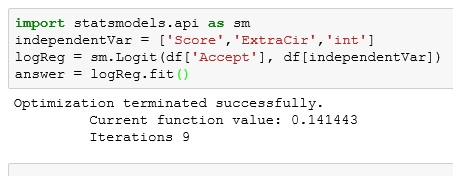The summary() function gives us a nice chart of our resultsIf you are a stats person, you can appreciate this. But for what we need, let us focus on our coef.remember our formula from above: y = c + c1*Score + c2*ExtraCir

Let’s build a function that solves for it.

Now let us see how a student with a Score of 1125 and a ExCir of 1 would fair.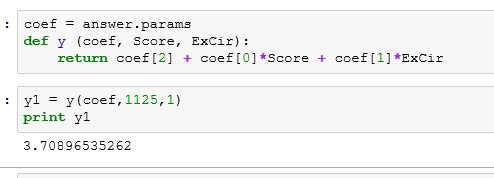okayyyyyy. So does 3.7089 mean they got in?????

Let’s take a quick second to think about the term logistic. What does it bring to mind?

Logarithms!!!

Okay, but our results equation was linear — y = c+ c1*Score + c2*ExCir

So what do we do.

So we need to remember y is a function of probability.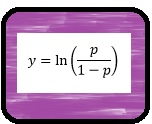So to convert y our into a probability, we use the following equationSo let’s import numpy so we can make use of e (exp() in Python)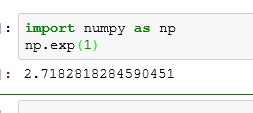Run our results through the equation. We get .97. So we are predicting a 97% chance of acceptance.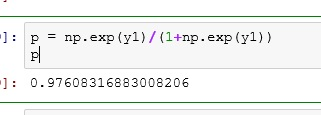Now notice what happens if I drop the test score down to 75. We end up with only a 45% chance of acceptance.If you enjoyed this lesson, click LIKE below, or even better, leave me a COMMENT.

# Python: Linear Regression

Regression is still one of the most widely used predictive methods. If you are unfamiliar with Linear Regression, check out my: Linear Regression using Excel lesson. It will explain the more of the math behind what we are doing here. This lesson is focused more on how to code it in Python.

We will be working with the following data set: Linear Regression Example File 1

## Import the data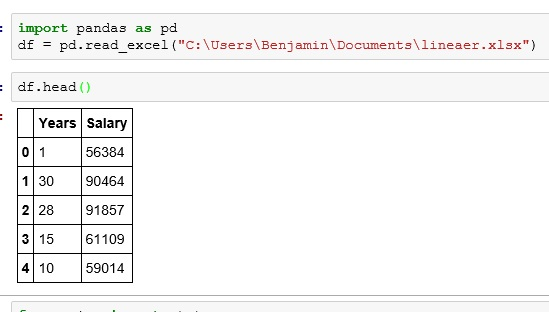What we have is a data set representing years worked at a company and salary.

## Let’s plot it

Before we go any further, let’s plot the data.

Looking at the plot, it looks like there is a possible correlation.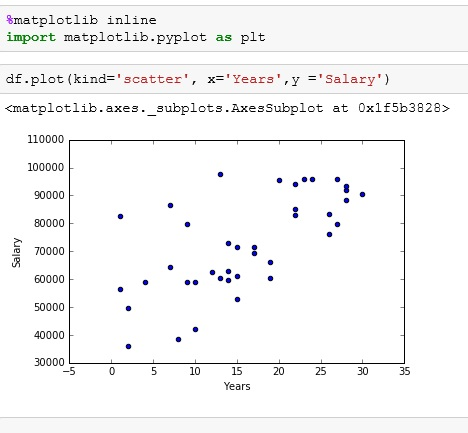## Linear Regression using scipy

scipy library contains some easy to use maths and science tools. In this case, we are importing stats from scipy

the method stats.linregress() produces the following outputs: slope, y-intercept, r-value, p-value, and standard error.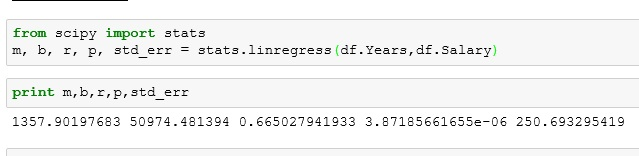I set slope to m and y-intercept to b: so we match the linear formula y = mx+b

Using the results of our regression, we  can create an easy function to predict a salary. In the example below, I want to predict the salary of a person who has been working there 10 years.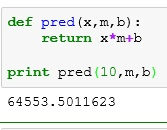Our p value is nice and low. This means our variables do have an effect on each other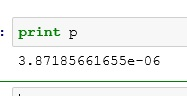Our standard error is 250, but this can be misleading based on the size of the values in your regression. A better measurement is r squared. We find that by squaring our r outputR squared runs from 0 (bad) to 1 (good). Our R squared is .44. So our regression is not that great. I prefer to keep a r squared value at least .6 or above.

## Plot the Regression Line

We can use our pred() function to find the y-coords needed to plot our regression line.

Passing pred() a x value of 0 I get our bottom value. I pass pred() a x value of 35 to get our top value.

I then redo my scatter plot just like above. Then I plot my line using plt.plot().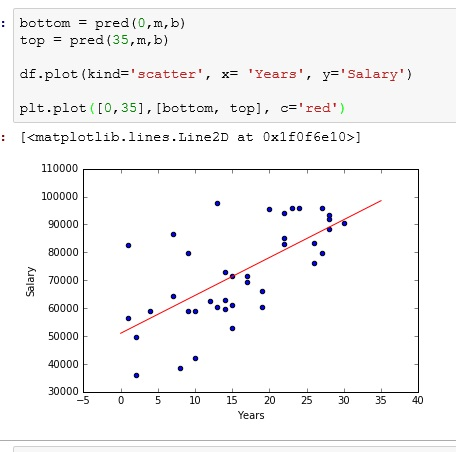If you enjoyed this lesson, click LIKE below, or even better, leave me a COMMENT.

# Python: Co-variance and Correlation

In this lesson, I will using data from a CSV file. You can download the file here: heightWeight

If you do not know how to import CSV files into Python, check out my lesson on it first: Python: Working with CSV Files

## The Data

The data set includes 20 heights (inches) and weights(pounds). Given what you already know, you could tell me the average height and average weight. You could tell me medians, variances and standard deviations.But all of those measurements are only concerned with a single variable. What if I want to see how height interacts with weight? Does weight increase as height increases?

**Note while there are plenty of fat short people and overly skinny tall people, when you look at the population at large, taller people will tend to weigh more than shorter people. This generalization of information is very common as it gives you a big picture view and is not easily skewed by outliers.

## Populate Python with Data

The first thing we are going to focus on is co-variance. Let’s start by getting our data in Python.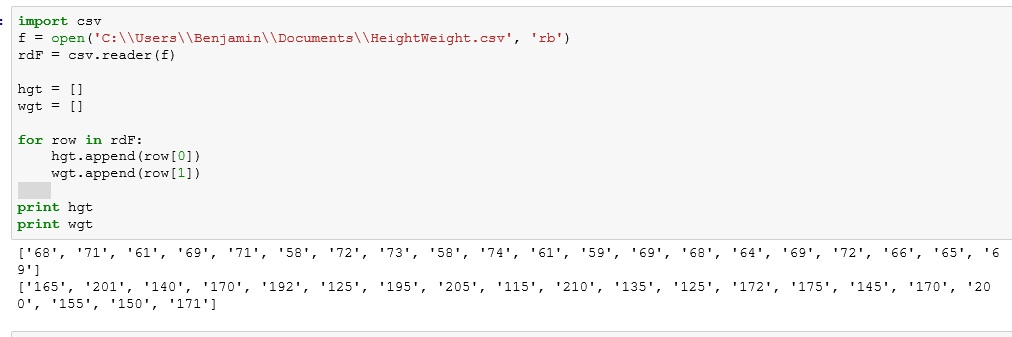Now there is a small problem. Our lists are filled with strings, not numbers. We can’t do calculations on strings.

We can fix this by populating converting the values using int(). Below I created 2 new lists (height and weight), created a for loop counting up to number of values in our lists : range(len(hgt)). Then I filled the new lists using lst.append(int(value))**Now I know I could have resolved this in fewer steps, but this is a tutorial, so I want to provide more of a walk through.

## Co-variance

Co-variance tells us how much two variables disperse from the mean together. There are multiple ways to find co-variance, but for me, using a dot product approach has always been the simplest.

For those unfamiliar with dot product. Imagine I had 2 lists (a,b) with 4 elements each. The dot product with calculated as so: a*b+a*b+a*b+a*b

Here is how it works:

If I take the individual variance of height and weight and they are both positive or negative – the product will be positive. – both variables are moving in the same direction

One positive and one negative will be negative. The variables are moving in different directions

One you add them all up, a positive number will mean that overall, you variables seem to have a positive co-variance (if a goes up, b goes up – if a goes down, b goes down)

If the final result is negative, you have negative co-variance (if a goes up, b goes down – if a goes down, b goes up)

Okay, let’s program this thing

** we will be using numpy’s mean() – mean and dot() – dot product methods and corrcoef() – correlation coefficient

First we need to find the individual variances from mean for each list

I create a function called ind_var that uses a list comprehension to subtract the mean from each element in the list.Now, let’s change out the print statement for a return, because we are going to be using this function inside another function.## Co-variance function

Now let’s build the co-variance function. Here we are taking the dot product of the variances of each element of height and weight. We then divide the result by the N-1 (the number of elements – 1 : the minus 1 is due to the fact we are dealing with sample data not population)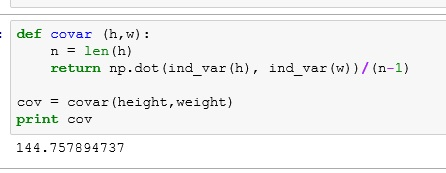So what were are doing:

• Take the first height (68 inches) and subtract the mean (66.8) from it (1.2)
• Take the first weight (165 lbs) and subtract the mean(165.8)  from it (-0.8)
• We then multiply these values together (-0.96)
• We repeat this for each element
• Divide by 19 (20-1)

Our result is 144.75789  – a positive co-variance. So when height goes up – weight goes up, when height goes down – weight goes down.

But what does 144 mean? Not much unfortunately. The co-variance doesn’t relate any information as to what units we are working with. 144 miles is a long way, 144 cm not so much.

## Correlation

So we have another measurement known as correlation. A very basic correlation equation divides out the standard deviation of both height and weight. The result of a correlation is between 1 and -1. With -1 being perfect anti-correlation and 1 being perfect correlation. 0 mean no correlation exists.

With my equation get 1.028  – more than one. This equation is simplistic and prone to some error.numpy’s corrcoef() is more accurate. It shows us a correlation matrix. Ignore the 1’s – they are part of what is known as the identity. Instead look at the other numbers = 0.97739.  That is about as close to one as you will ever get in reality. So even if my equation is off, it isn’t too far off.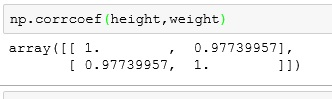Now just to humor me. Create another list to play with.Let’s run this against height in my correlation functionRun these value through the more accurate corrcoef() . This will show my formula is still a bit off, but for the most part, it is not all that bad.If you enjoyed this lesson, click LIKE below, or even better, leave me a COMMENT.

# Python: Fun with Central Tendency

Now numpy provides easy functions for finding central tendency – you can find them in my Numpy Part II lesson: Python: Numpy Part II.

But we have learned enough about Python so far, that maybe it would be more fun to build our own functions. In this lesson we are going to build our own statistics library with mean, median, mode, and quantile

## Our Data## Mean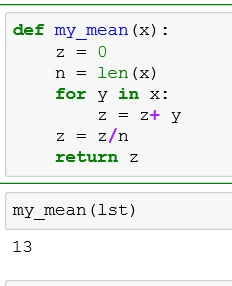or even easier: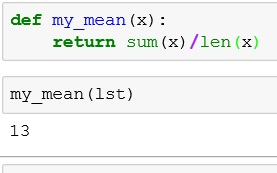## Median

Remember with median – if your data contains an odd number of elements (n%2==1), you just take the middle value. However, if your data contains and even number of elements (n%2==0) then you add the two middle numbers and divide by 2.

We handle that through the use of an if statement in our function.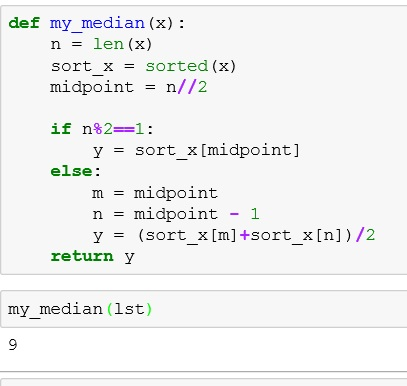## Mode

For mode, we want to find the most common element in the list. For this task, I will import Counter from collections.

d.most_common() returns each unique element and the number of times it appears

d.most_common(1) returns the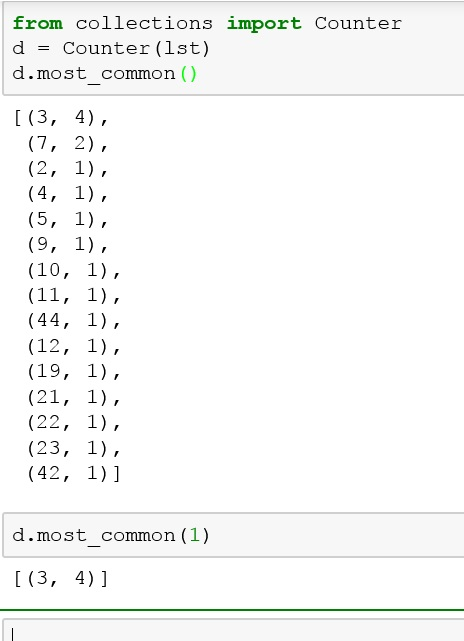or in function form: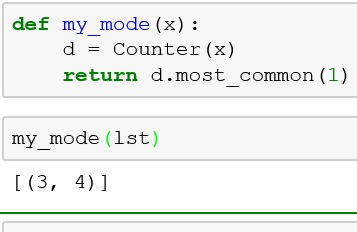In my numpy part II lesson I use a more elegant solution, but I want you to see that there is always more than one way to do something in Python.

## Quantile

Quantiles are cut points in set of data. They can represent the bottom ten percent of the data or the top 75% or any % from 0 to 100.

In my solution, I am adding a slicing argument to the sorted() function. Below shows all elements up to (but not including) index 4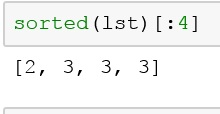quantile function:If you enjoyed this lesson, click LIKE below, or even better, leave me a COMMENT.